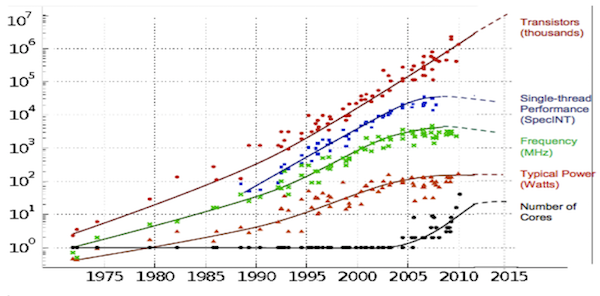# OTODSCINOs: The Root Cause of Slow Neural Net Training

I’ve been interested in the fundamentals of numerical optimization ever since dropping in Stephen Boyd’s “Convex Optimization” class in Stanford years ago. Theory developed in this field can be used to answer questions faced by today’s practitioners, in particular, the question of — “Why does it take so long to train neural nets?”

Conv nets trained in the 80s on handwritten digits took about 1 week to train on hardware of the day. Today’s research conferences want your methods demonstrated on ImageNet-sized datasets, which also take a week on hardware of the day (a single high end GPU).

One factor for stalling wall-clock times is psychological. Thirst for knowledge is unquenchable so researchers will keep increasing computational load until it takes too long. Some engineers at Google use 10 PFlop TPU pods to train exotic models on MNIST. Clearly, a creative person will find a way to exhaust any amount of computation available to them.

However, there are also fundamental factors that are keeping training times high. Consider the following code to train a neural net:

`for i in range(3000000): params-=gradient(params) if objective(params)<0.01: break`

Even though the number of available transistors has been growing exponentially, if you have to do 3 million iterations in a sequence, at some point extra transistors stop helping and you are limited by the single thread performance.

Single thread performance has not been doing great. Consider this chart:C Moore, Data Processing in Exa-Scale-Class Computer Systems, Salishan, April 2011

Serial performance hit a peak around 2007 and has been flagging since. In practice I found that training some Atari RL models was faster on my 3 year old laptop than on the latest Intel Xeon chip.

To get around poor single thread performance we need to figure out ways to reduce the number of iterations in the training loop.

The number of iterations has been kept high because of the following 3 OTODSCINOs (Obstacles TO Decreasing Serial Complexity In Nonlinear Optimization)

# OTODSCINO 1: amount of non-linearity

Consider the following landscape of a non-linear optimization problem:

Because gradient descent works on local information, there’s a certain number of steps it needs to take until it can even “see” the minimum.

Non-linearity can be characterized by interactions between components of the objective function, and as you add more layers to a network, it increases potential for interactions.

Take a toy example, multiply a few random matrices together and try to minimize the norm of the result by tweaking arbitrary entries a,b:

Even for this purely linear neural network, the optimization problem becomes nonlinear with more layers.

To summarize:

# OTODSCINO 2: Local condition number

Consider the following minimization problem:

This is a linear estimation problem so OTODSCINO 1 doesn’t apply. However, it’s still difficult for gradient descent because gradient doesn’t point towards the minimum. Gradient descent for this problem would follow a characteristic zig-zag path:

There’s a quantity that measures how hard such a problem is for gradient descent — “condition number.” It is the ratio of largest diameter to smallest diameter and it is 10 for the problem above.

The number of steps needed to get close to the minimum grows as O(condition number):

Number of steps grows as O(k).

Condition number 1: minimization takes 1 step.

Condition number 10: minimization takes 10 steps.

The neural network’s optimization surface is badly conditioned (empirical evaluation in this paper), and adding parameters makes conditioning worse.

To summarize:

# OTODSCINO 3: Amount of gradient noise

Neural net optimization uses stochastic gradient descent rather than gradient descent. Our already bad estimates of direction are further diluted by noise. Here’s an example of trying to minimize Rosenbrock’s function with and without the noise:

Noise increases as you add dimensions to parameter space. It is easiest to see for Gaussian normal noise. Root-mean-squared error introduced by d-dimensional noise grows approximately as sqrt(d)

For derivation, see here.

This last OTODSCINO has some hope — even though noise grows with additional dimensions, we can use our exponentially growing pool of transistors to compute estimates in parallel and average them together.

From weak law of large numbers, we know that errors shrinks as sqrt(n) where n is the number of samples.

Having square root both in noise and average formulas gives an easy to remember rule of thumb — to avoid extra noise making things worse, you can increase batch size at the same rate as number of parameters.

To summarize:

But with extra parallel compute, you can negate this:

To drive down wall-clock times we need to chip on the three OTODSCINOs. The first obstacle can be addressed by using “less nonlinear” parameterizations of neural nets. Resnet and ReLU activations are examples of advances in that area. The second obstacle can be mitigated by adapting advanced methods from linear estimation to neural networks, like KFAC. The third obstacle needs software engineering to make it easier to use an ensemble of many devices. Finally, all three obstacles can be mitigated by next-generation hardware that will use transistors more efficiently for deep learning. A recent survey found 45 startups in this space.

To follow along with more South Park Commons members’ research and projects, sign up for the SPC email newsletter.

--

--